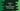# How to use logical operator with strings in Python## How to use logical operator with strings in Python:

We can use the logical operators with strings in Python. We have three logical operators available: AND, OR and NOT. If you are familiar with these operators, you can use these to check for empty strings in Python. An empty string is treated as False and a non-empty string as True if we use these with logical operators.

In this post, I will show you how to use strings with logical operators in Python with different examples.

### Logical operators:

The logical operators are used with multiple boolean values. We can also use statements if the result of that is boolean.

Following three logical operators are available in Python:

1. Logical AND. The logical AND operator returns True if both values are True. Otherwise it returns False.
2. Logical OR. The logical OR operator returns True if any of the value is True. Else, it returns False.
3. Logical NOT. The logical NOT operator reverses the result. For example, if we pass True, it returns False and for False, it returns True.

Let’s take a look at the below example:

``````print('True and True', True and True)
print('True and False', True and False)
print('False and True', False and True)
print('False and False', False and False)
print()
print('True or True', True or True)
print('True or False', True or False)
print('False or True', False or True)
print('False or False', False or False)
print()
print('not True', not True)
print('not False', not False)``````

This program is printing the result of all cases for and, or and not logical operators.

If you run this program, it will print the below result:

``````True and True True
True and False False
False and True False
False and False False

True or True True
True or False True
False or True True
False or False False

not True False
not False True``````

As you can see here, for and, it is True if both are True. For or, it is True if any one of the values is True and for not, it returns the reverse.

### Logical operators with strings:

Let’s try it with strings. As I have told you before, an empty string is translated to False and a non-empty string is translated to True.

#### AND with strings:

Let’s try a non-empty string with True/False and AND operator:

``````print('True and \'Hello World\':', True and 'Hello World')
print('\'Hello World\' and True:', 'Hello World' and True)
print('False and \'Hello World\':', False and 'Hello World')
print('\'Hello World\' and False:', 'Hello World' and False)``````

It will print:

``````True and 'Hello World': Hello World
'Hello World' and True: True
False and 'Hello World': False
'Hello World' and False: False``````

If there is False, it prints False. If there is True, it prints True or the string.

Now, let’s try it with an empty string:

``````print('True and \'\':', True and '')
print('\'\' and True:', '' and True)
print('False and \'\':', False and '')
print('\'\' and False:', '' and False)``````

It will print:

``````True and '':
'' and True:
False and '': False
'' and False: ``````

For the first two and the last line, it returned an empty string or False. With and, all results are False in this example.

#### OR with strings:

Let’s try the above example with OR:

``````print('True or \'Hello World\':', True or 'Hello World')
print('\'Hello World\' or True:', 'Hello World' or True)
print('False or \'Hello World\':', False or 'Hello World')
print('\'Hello World\' or False:', 'Hello World' or False)``````

It will print:

``````True or 'Hello World': True
'Hello World' or True: Hello World
False or 'Hello World': Hello World
'Hello World' or False: Hello World``````

It returns True or the string.

Similarly, if we use an empty string:

``````print('True or \'\':', True or '')
print('\'\' or True:', '' or True)
print('False or \'\':', False or '')
print('\'\' or False:', '' or False)``````

It will print:

``````True or '': True
'' or True: True
False or '':
'' or False: False``````

#### NOT with strings:

Let’s use NOT with an empty and non-empty string:

``````print('not \'hello world\'', not 'hello world')
print('not \'\'', not '')``````

It will print:

``````not 'hello world' False
not '' True``````

For the non-empty string, it returns False and for the empty string it returns True.

### Combining the result with if-else statements:

We can combine the result of logical operators with if-else statements. For example:

``````str_1 = 'hello world'
str_2 = ''
str_3 = ''

if str_1:
print('str_1 is not empty')

if str_1 or str_2:
print('str_1 or str_2 is empty')

if str_2 and str_3:
print('str_2 and str_3 are not empty')
else:
print('str_2 and str_3 are empty')

if not str_2:
print('str_2 is empty')``````

It will print:

``````str_1 is not empty
str_1 or str_2 is empty
str_2 and str_3 are empty
str_2 is empty``````

As the return value of logical operator is a boolean value, we can use it with an if-else statement.